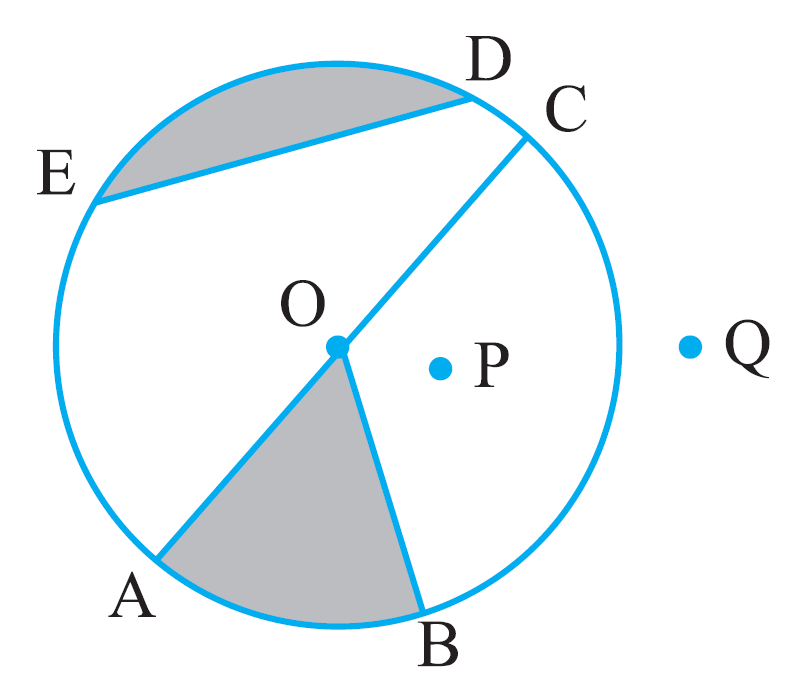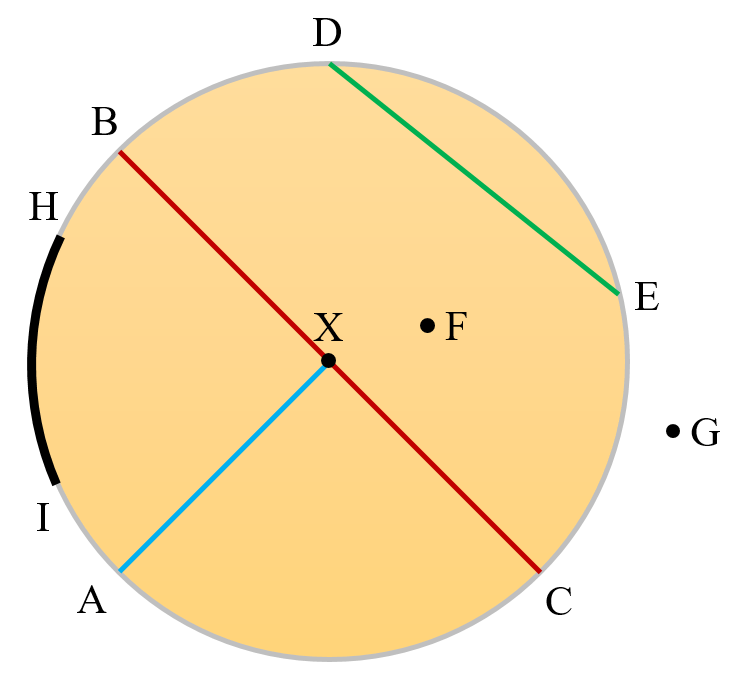# vs.eyeandcontacts.com

## Chapter 4 Basic Geometrical Ideas Exercise 4.6

Question 1: From the figure, identify:a) the centre of circle
c) a diameter
d) a chord
e) two points in the interior
f) a point in the exterior
g) a sector
h) a segment

a) O is the centre of the circle.
b) Line segment OA, line segment OB, line segment OC are the three radii.
c) Line segment AC is the diameter.
d) A chord is Line segment ED.
e) O and P are two points in the interior.
f) Q is the point in the exterior.
g) A sector is OAB (shaded part).
h) A segment is ED (shaded part).

Question 2:
a) Is every diameter of a circle also a chord?
b) Is every chord of a circle also a diameter?

a) Yes, every diameter of a circle is also a chord. It is also known as the longest chord of a circle.
b) No, every chord of a circle is not a diameter.

Question 3: Draw any circle and mark
a) its centre
c) a diameter
d) a sector
e) a segment
f) a point in its interior
g) a point in its exterior
h) an arca) X is the centre of the circle.
b) Line segment XA is the radius of the circle.
c) Line segment BC is the diameter of the circle.
d) A sector is XAC.
e) A segment is DE.
f) F is the point in the interior of the circle.
g) G is the point in the exterior of the circle.
h) An arc is arc HI.

Question 4: Say true or false:
a) Two diameters of a circle will necessarily intersect.
b) The centre of a circle is always in its interior.